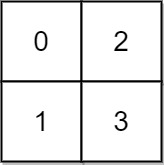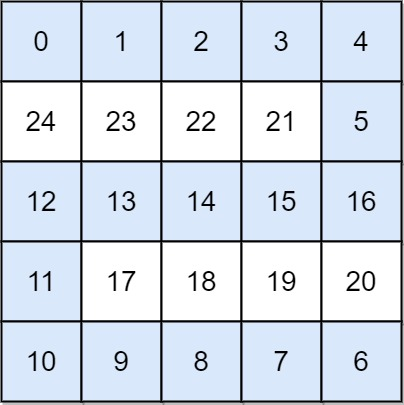## Algorithm

Problem Name: 778. Swim in Rising Water

You are given an `n x n` integer matrix `grid` where each value `grid[i][j]` represents the elevation at that point `(i, j)`.

The rain starts to fall. At time `t`, the depth of the water everywhere is `t`. You can swim from a square to another 4-directionally adjacent square if and only if the elevation of both squares individually are at most `t`. You can swim infinite distances in zero time. Of course, you must stay within the boundaries of the grid during your swim.

Return the least time until you can reach the bottom right square `(n - 1, n - 1)` if you start at the top left square `(0, 0)`.

Example 1:```Input: grid = [[0,2],[1,3]]
Output: 3
Explanation:
At time 0, you are in grid location (0, 0).
You cannot go anywhere else because 4-directionally adjacent neighbors have a higher elevation than t = 0.
You cannot reach point (1, 1) until time 3.
When the depth of water is 3, we can swim anywhere inside the grid.
```

Example 2:```Input: grid = [[0,1,2,3,4],[24,23,22,21,5],[12,13,14,15,16],[11,17,18,19,20],[10,9,8,7,6]]
Output: 16
Explanation: The final route is shown.
We need to wait until time 16 so that (0, 0) and (4, 4) are connected.
```

Constraints:

• `n == grid.length`
• `n == grid[i].length`
• `1 <= n <= 50`
• `0 <= grid[i][j] < n2`
• Each value `grid[i][j]` is unique.

## Code Examples

### #1 Code Example with Javascript Programming

```Code - Javascript Programming```

``````
const swimInWater = function(grid) {
let n = grid.length;
let low = grid,
hi = n * n - 1;
while (low < hi) {
let mid = low + Math.floor((hi - low) / 2);
if (valid(grid, mid)) hi = mid;
else low = mid + 1;
}
return low;
};

function valid(grid, waterHeight) {
let n = grid.length;
const visited = Array.from(new Array(n), el => new Array(n).fill(0));
const dir = [-1, 0, 1, 0, -1];
return dfs(grid, visited, dir, waterHeight, 0, 0, n);
}
function dfs(grid, visited, dir, waterHeight, row, col, n) {
visited[row][col] = 1;
for (let i = 0; i < 4; ++i) {
let r = row + dir[i],
c = col + dir[i + 1];
if (
r >= 0 &&
r < n &&
c >= 0 &&
c < n &&
visited[r][c] == 0 &&
grid[r][c] <= waterHeight
) {
if (r == n - 1 && c == n - 1) return true;
if (dfs(grid, visited, dir, waterHeight, r, c, n)) return true;
}
}
return false;
}
``````
Copy The Code &

Input

cmd
grid = [[0,2],[1,3]]

Output

cmd
3

### #2 Code Example with Python Programming

```Code - Python Programming```

``````
class Solution:
def swimInWater(self, grid):
heap, res, n, visited = [(grid, 0, 0)], 0, len(grid), set()
while True:
d, i, j = heapq.heappop(heap)
if d > res: res = d
if i == j == n - 1: return res
for x, y in ((i - 1, j), (i + 1, j), (i, j - 1), (i, j + 1)):
if 0 <= x < n and 0 <= y < n and (x, y) not in visited:
heapq.heappush(heap, (grid[x][y], x, y))
``````
Copy The Code &

Input

cmd
grid = [[0,2],[1,3]]

Output

cmd
3